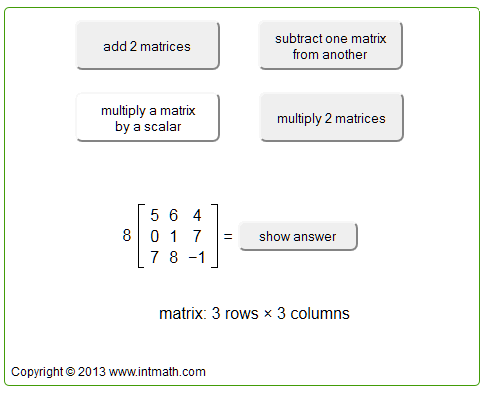Search IntMath
Close

# Updated matrix interactive

By Murray Bourne, 02 Oct 2013

Many students get quite lost when trying to figure out how matrices work, especially matrix multiplication. It's easy for the uninitiated to head off along the wrong row, or the wrong column. And it's frustrating when one small error early on can mean the whole thing is incorrect.

While math teachers (and/or the system) continue to require students to do these things on paper, I hope this applet helps students to see how each operation works.

(This is actually an update of an applet I've had on the site for a while. I'm in the process of replacing the Flash-based interactives in IntMath so they work on mobile devices. Technology never stands still...)

The new applet (or "mathlet" as some people like to call them) can be found here: Add, Subtract and Multiply matrices interactive.

In this interactive, you can investigate how addition, subtraction, scalar multiplication and multiplication of 2 matrices all work. You can step through each one in order to see how each number in the matrices is used, and where the answer matrix comes from.

Here are some screen shots:

Scalar multiplication:Matrix multiplication:

## For the geeks

The applet now uses jQuery. I hoped it would be quite straightforward to convert my earlier Flash-based applet, but it wasn't. Even though Flash's Actionscript is close to javascript, most of it had to be re-written.

### Comment Preview

HTML: You can use simple tags like <b>, <a href="...">, etc.

To enter math, you can can either:

1. Use simple calculator-like input in the following format (surround your math in backticks, or qq on tablet or phone):
a^2 = sqrt(b^2 + c^2)
(See more on ASCIIMath syntax); or
2. Use simple LaTeX in the following format. Surround your math with $$ and $$.
$$\int g dx = \sqrt{\frac{a}{b}}$$
(This is standard simple LaTeX.)

NOTE: You can mix both types of math entry in your comment.